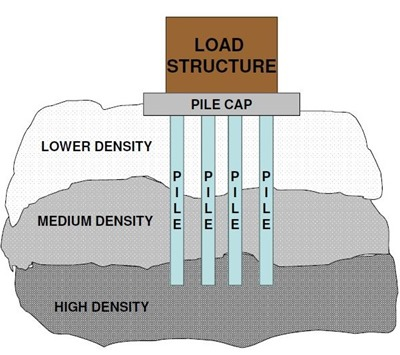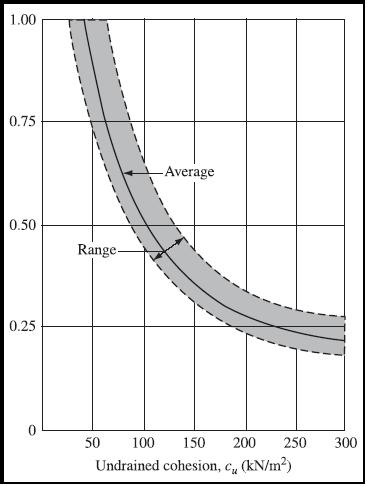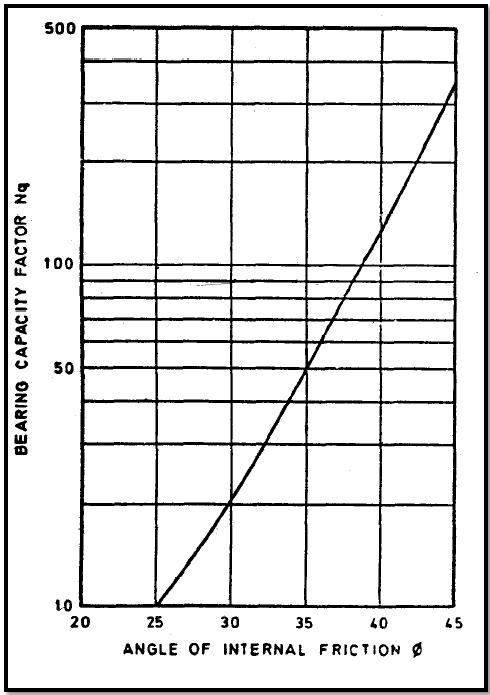# HOW TO CALCULATE PILE LOAD CAPACITY? (STATIC ANALYSIS)

The ultimate bearing capacity of a pile is the maximum load which it can carry without failure or excessive settlement of the ground.

The bearing capacity of a pile depends primarily on 3 factors as given below,

1. Type of soil through which pile is embedded
2. Method of pile installation
3. Pile dimension (cross section & length of pile)

While calculating pile load capacity for cast in situ concrete piles, using static analysis, we need to use soil shear strength parameter and dimension of pile.## Load Carrying Capacity of Pile Using Static Analysis

The pile transfers the load into the soil in two ways. Firstly, through the tip-in compression, termed as “end-bearing” or “point-bearing”; secondly, by shear along the surface termed as “skin friction”.

### Load carrying capacity of cast in-situ piles in cohesive soil

The ultimate load carrying capacity (Qu) of pile in cohesive soils is given by the formula given below, where the first term represents the end bearing resistance (Qb) and the second term gives the skin friction resistance (Qs).Where,

Qu = Ultimate load capacity, kN

Ap = Cross-sectional area of pile tip, in m2

Nc = Bearing capacity factor, may be taken as 9

αi = Adhesion factor for the ith layer depending on the consistency of soil. It depends upon the undrained shear strength of soil and may be obtained from the figure given below.Variation of alpha with cohesion

ci = Average cohesion for the ith layer, in kN/m2

Asi = Surface area of pile shaft in the ith layer, in m2

A minimum factor of safety of 2.5 is used to arrive at the safe pile load capacity (Qsafe) from ultimate load capacity (Qu).

Qsafe = Qu/2.5

### Load carrying capacity of cast in-situ piles in cohesion less soil

The ultimate load carrying capacity of pile, “Qu”, consists of two parts. One part is due to friction, called skin friction or shaft friction or side shear denoted as “Qs” and the other is due to end bearing at the base or tip of the pile toe, “Qb”.

The equation given below is used to calculate the ultimate load carrying capacity of pile.Where,

Ap = cross-sectional area of pile base, m2

D = diameter of pile shaft, m

γ = effective unit weight of the soil at pile tip, kN/m3

Nγ= bearing capacity factor

Nq = bearing capacity factor

Φ = Angle of internal friction at pile tip

PD = Effective overburden pressure at pile tip, in kN/m2

K = Coefficient of earth pressure applicable for the ith layer

PDi = Effective overburden pressure for the ith layer, in kN/m2

δi = Angle of wall friction between pile and soil for the ith layer

Asi = Surface area of pile shaft in the ith layer, in m2

The first term is the expression for the end bearing capacity of pile (Qb) and the second term is the expression for the skin friction capacity of pile (Qs).

A minimum factor of safety of 2.5 is used to arrive at the safe pile capacity (Qsafe) from ultimate load capacity (Qu).

Qsafe = Qu / 2.5

#### Important Notes to remember

• The value of bearing capacity factor Nq is obtained from the figure given below.bearing factor value
• The value of bearing capacity factor Nγ is computed using the equation given below.• For driven piles in loose to dense sand with φ varying between 300to 400 , ki values in the range of 1 to 1.5 may be used.
• δ, the angle of wall friction may be taken equal to the friction angle of the soil around the pile stem.
• The maximum effective overburden at the pile base should correspond to the critical depth, which may be taken as 15 times the diameter of the pile shaft for φ ≤ 300and increasing to 20 times for φ ≥ 400
• For piles passing through cohesive strata and terminating in a granular stratum, a penetration of at least twice the diameter of the pile shaft should be given into the granular stratum.
9 Comments
1.2.3.4.5.6.7.8.9.